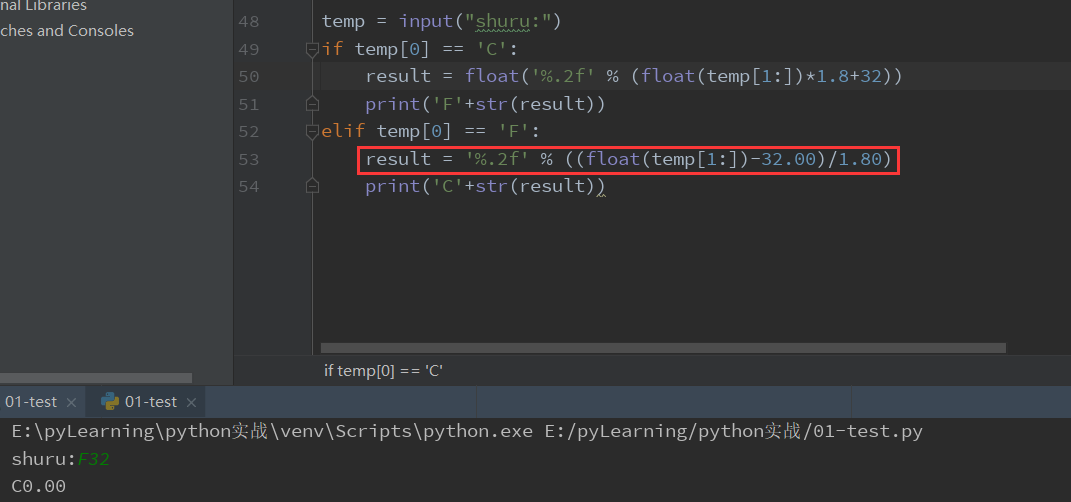2018-08-13 15:10

# 初学Python，温度转换程序

5

Python编程练习温度转换

``````     C = ( F - 32 ) / 1.8

F = C * 1.8 + 32
``````

• 输入输出的摄氏度采用大写字母C开头，温度可以是整数或小数，如：C12.34指摄氏度12.34度；
• 输入输出的华氏度采用大写字母F开头，温度可以是整数或小数，如：F87.65指摄氏度87.65度；
• 不考虑异常输入的问题，输出保留小数点后两位；
• 使用input()获得测试用例输入时，不要增加提示字符串

``````emp = input()
if temp == 'C':
result = float('%.2f' % (float(temp[1:])*1.8+32))
print('F'+str(result))
elif temp == 'F':
result = float('%.2f' % ((float(temp[1:])-32)/1.8))
print('C'+str(result))
``````

``````输出：C0.0
``````

• 点赞
• 写回答
• 关注问题
• 收藏
• 复制链接分享
• 邀请回答

#### 2条回答

• ``````temp = "F32"
if temp == 'C':
result = float('%.2f' % (float(temp[1:])*1.8+32))
print('F{:.2f}'.format(result))
elif temp == 'F':
result = float('%.2f' % ((float(temp[1:])-32)/1.8))
print('C{:.2f}'.format(result))
``````
点赞 2 评论 复制链接分享
• `````` temp = input("shuru:")
if temp == 'C':
result = float('%.2f' % (float(temp[1:])*1.8+32))
print('F'+str(result))
elif temp == 'F':
result = '%.2f' % ((float(temp[1:])-32.00)/1.80)
print('C'+str(result))
``````这条代码你原来写法是将 %.2f （此时已经是0.00了）然后你又加强制转换float（）因为小数点后面是两个0 所以自动变成0.0只是说是浮点型 而不考虑小数位数，删去float即可
代码如上。
这样就ok了

点赞 2 评论 复制链接分享